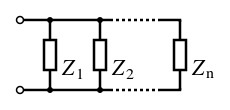# Algebraic Electronics!Consider a network Q consisting of 2015 distinct parallel impedances (in ohms) $Z_{1}, Z_{2}, \ldots, Z_{2015}$, such that each of these individual values satisfy the equation $(Z-2)^{2015} = 2^{2017}$.

Determine the total impedance (in ohms) of an impedance network consisting of 2015 Q networks in series.

×